# Project 1 Phase 1 22 C 021 CS

• Slides: 9
Download presentationProject 1 : Phase 1 22 C: 021 CS II Data StructuresProject 1 Plan • Part I (for week 1) – Turn the my. List. Graph class into a generic class. – Implement clustering. Coefficient(v) and clustering. Coefficient() – Add an additional data member to the my. List. Graph class called “locations” to keep track of an array of points. – Add the constructor: my. List. Graph(n, d, p) to my. List. Graph.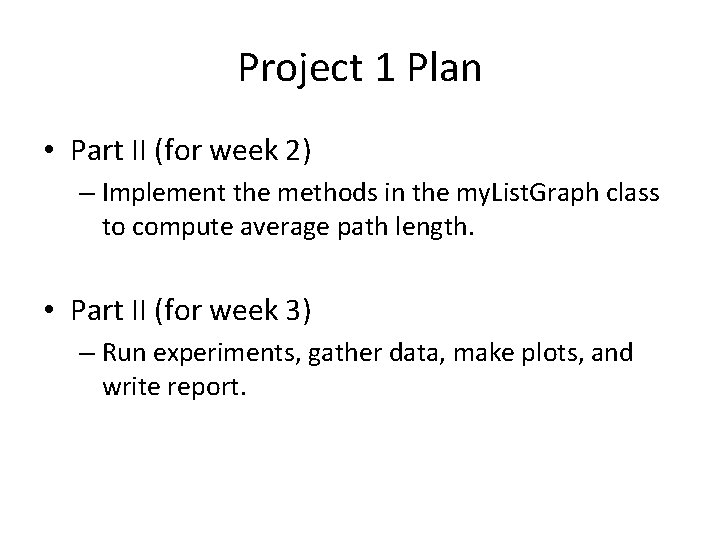Project 1 Plan • Part II (for week 2) – Implement the methods in the my. List. Graph class to compute average path length. • Part II (for week 3) – Run experiments, gather data, make plots, and write report.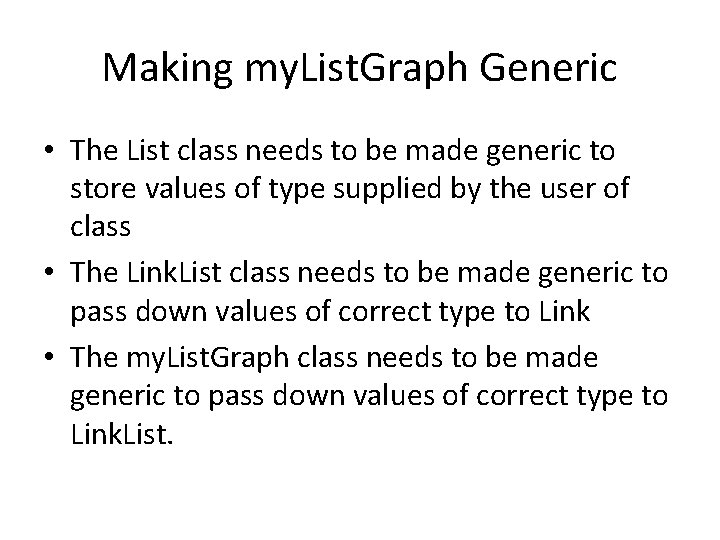Making my. List. Graph Generic • The List class needs to be made generic to store values of type supplied by the user of class • The Link. List class needs to be made generic to pass down values of correct type to Link • The my. List. Graph class needs to be made generic to pass down values of correct type to Link. List.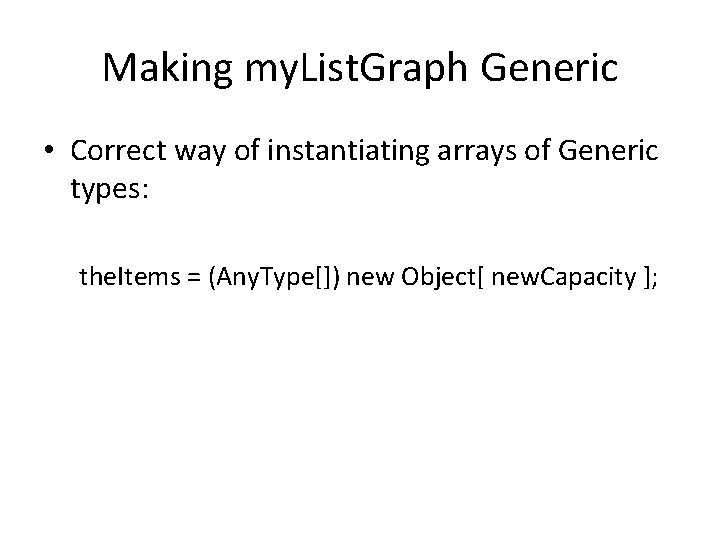Making my. List. Graph Generic • Correct way of instantiating arrays of Generic types: the. Items = (Any. Type[]) new Object[ new. Capacity ];Code to Test Generic my. List. Graph • Will soon be available on class webpage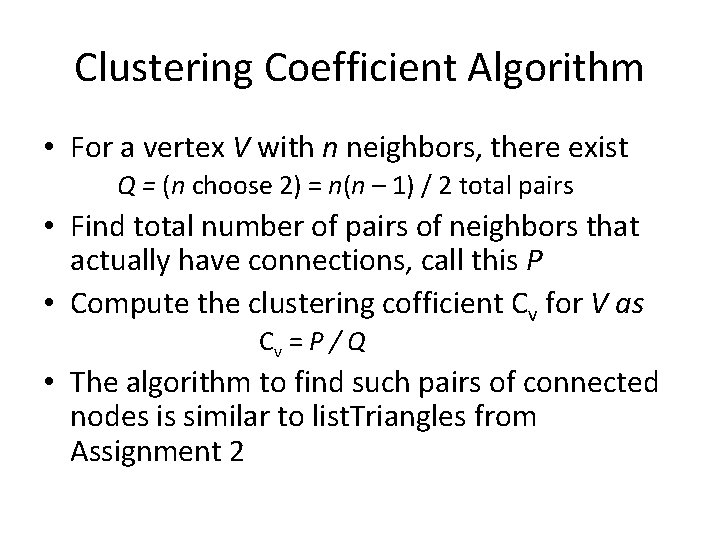Clustering Coefficient Algorithm • For a vertex V with n neighbors, there exist Q = (n choose 2) = n(n – 1) / 2 total pairs • Find total number of pairs of neighbors that actually have connections, call this P • Compute the clustering cofficient Cv for V as Cv = P / Q • The algorithm to find such pairs of connected nodes is similar to list. Triangles from Assignment 2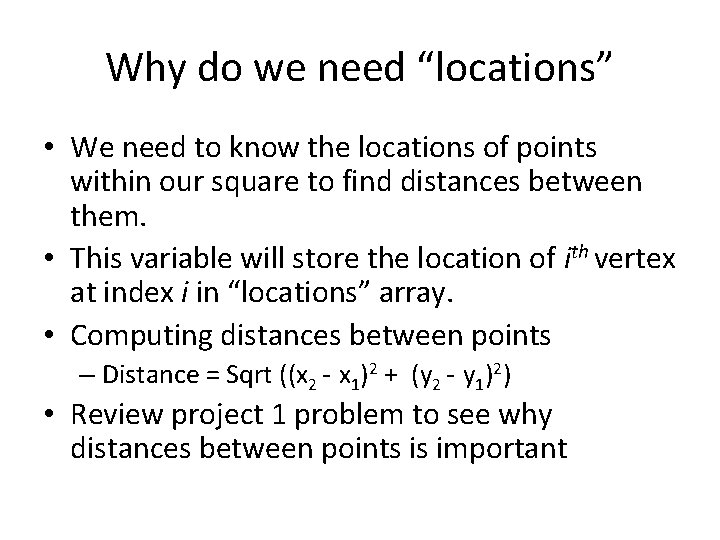Why do we need “locations” • We need to know the locations of points within our square to find distances between them. • This variable will store the location of ith vertex at index i in “locations” array. • Computing distances between points – Distance = Sqrt ((x 2 - x 1)2 + (y 2 - y 1)2) • Review project 1 problem to see why distances between points is importantUsing Probability factor • While generating a graph, probably factor p determines if we connect to another of our neighbors or to a non-neighbor • To use this factor, generate a floating random number and compare it with p – If the generated number is less than p, connect to a non-neighbor – Otherwise, connect to your neighbor